# Voltage source and current source

PhysicsTest
TL;DR Summary
Want to understand difference between voltage and current source.
As per ohms law the current is generated based on voltage and resistance V=IR. So if 5v and 1k resistor the current 5mA flows in resistor. But if I take a current source, can I pump any amount of current to resistor? So, current drawn is not characteristic of resistor?

Mentor
One key concept for current sources is their "voltage compliance range". That means that the output of a current source will only be accurate over some range of output voltages.

So for example, if you have a 12V power supply for your current source circuit, the output compliance voltage will likely be something like 0V to 10V. If you put a big enough current through a big enough resistor so that the output voltage climbs above 10V, then your output current will drop from the expected value (you are "out of voltage compliance").

You can see why this is true if you look at typical current source circuits, with a high-side PNP transistor as the main control element, for example. Or a low-side current sink NPN transistor for the control element as shown in the following figure: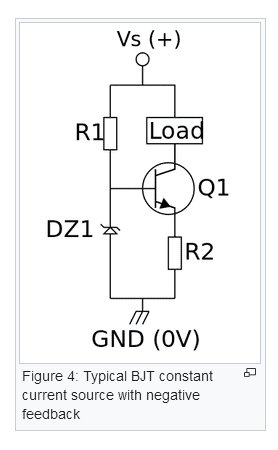https://en.wikipedia.org/wiki/Current_source

•hutchphd
Gold Member
Voltage and current sources are ideal circuit elements used to simplify circuit models. They don't exist IRL as perfect sources. Of course, a perfect voltage source will always, no matter what, create a defined voltage across it. Similar for a perfect current source.

You can model any voltage/current source as a perfect source with an associated internal resistance ##R_T##. Using the Thevenin/Norton models these could either be a perfect voltage source with a series resistance (impedance, really), or a perfect current source with a parallel resistance. Both models are equivalent according to those theorems.

You would calculate or measure the quality of a voltage source by examining how much does the terminal voltage difference change when you (the circuit) changes the current drawn from it. This metric ## \frac{\Delta V}{\Delta I} = R_T ## is just the Thevenin equivalent resistance. This is ## \frac{\partial V}{\partial I} = R_T ## if you know calculus. There is a dual case for current sources, constant current regardless of the voltage imposed on it's terminals. So, a perfect voltage source has ## \frac{\Delta V}{\Delta I} = R_T = 0##. A perfect current source has ## \frac{\Delta V}{\Delta I} = R_T = \infty##.

So, the distinction between voltage and current sources really comes down to whether the internal impedance is high or low (relative to the circuit it's connected to). Real world sources are somewhere between a voltage or a current source. For example the battery in your car is a good voltage source, but not perfect. That's why they have specifications for "cold cranking amps" for example. If it was perfect, CCR would be infinite.

•cabraham, hutchphd and berkeman
Gold Member
2022 Award
Summary:: Want to understand difference between voltage and current source.

As per ohms law the current is generated based on voltage and resistance V=IR. So if 5v and 1k resistor the current 5mA flows in resistor. But if I take a current source, can I pump any amount of current to resistor? So, current drawn is not characteristic of resistor?
Although what @berkeman says is true, I think it's a bit of an aside from your question since based on your question, it's clear that you need a simple fundamental description of IDEAL voltage and current sources of the kind used in beginner problems before you move on to the real world.

SO ... at the most simple level, a voltage source provides voltage without restriction but you're not allowed to short-circuit it because that would imply infinite current (Ohm's Law) and a current source provides current without limit but you're not allowed to have an open circuit across it because that would imply infinite voltage (Ohm's Law).

In answer to your question, yes a current source can pump any amount of current into a resistor (this is an ideal situation, not real world). Ideal resistors have no restriction on the amount of voltage across them (but not infinite) or the current through them (but not infinite).

Both @berkeman and @DaveE have given good discussions of real-world situations.

Last edited:
•cabraham, hutchphd and berkeman
Gold Member
It may be helpful to look at a V vs. I plot of 2-terminal devices. This is for all possible DC conditions.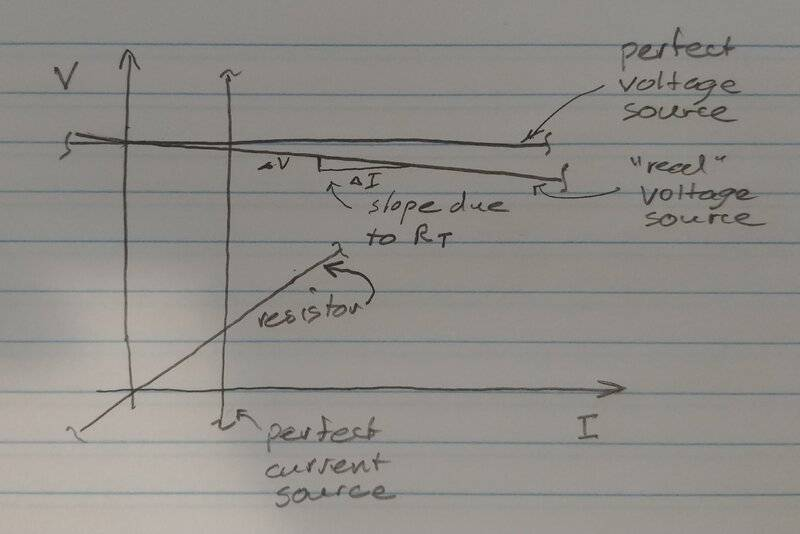Staff Emeritus
Or a real device that is both. A solar cell, like in a solar panel, acts like a current source for low voltages. I acts (almost) like a voltage source for low currents. So, depending on what your interest is, you can model a solar cell like an ideal constant current source or an ideal voltage source.

It is like saying that a line can be (almost) vertical and (almost) horizontal.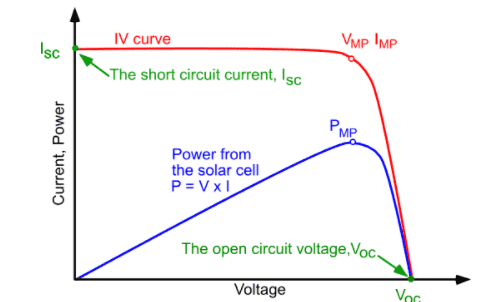Gold Member
Or a real device that is both. A solar cell, like in a solar panel, acts like a current source for low voltages. I acts (almost) like a voltage source for low currents. So, depending on what your interest is, you can model a solar cell like an ideal constant current source or an ideal voltage source.

It is like saying that a line can be (almost) vertical and (almost) horizontal.

View attachment 291781

This is a perfect example of why you may want to use a simplistic model for rough a analysis. A designer working at the low voltage - high current region would want to substitute a current source in a 1st order model. But that won't work for the guy designing at the high voltage - low current region, they"'ll want to use a voltage source and series resistor. Either way, a simple model is a great first step, and is often all you really need.

Staff Emeritus
2022 Award
As per ohms law the current is generated based on voltage and resistance V=IR. So if 5v and 1k resistor the current 5mA flows in resistor. But if I take a current source, can I pump any amount of current to resistor? So, current drawn is not characteristic of resistor?
I think you're asking whether Ohm's law holds when using current sources, to which the answer is yes, it does.
Pump an amount of current i through the resistor, however you achieve that current, and you will find that the voltage across the resistor is equal to i times r.

As an exercise, do the following on some paper. Take a voltage source of 5 V and put it in series with a 5 K ohm resistor. This will be your non-ideal current source. Then put both of these in series with a resistive load. Vary the resistance of the load a few times between 50 ohms and 450 ohms. You'll find that the current through the load remains within 5% of 1 mA, which is why this is a current source. In addition, find the voltage drop across both resistors each time you change the load. You'll find that the total voltage drop remains 5V and that the current times the resistance of the load always equals the voltage drop across it.

The thing about current sources that they don't tell you about is how they actually work, which is both good and bad. Good because you don't have to worry about it, but bad because it can confuse you and think that certain things don't seem to apply to them, like Ohm's law. The same is actually true for voltage sources, but the amount of variation in the voltage from the source is generally very small for most circuits, so we don't tend to worry about it very much in early EE courses.

If you're curious about voltage sources, again take some pen and paper and put an ideal voltage source in series with a 0.2 ohm resistor and then connect various loads to it. The voltage source and resistor form a non-ideal voltage source. As you vary the resistance of the load, you'll see that the voltage across the load remains very, very close to 5 V unless you make the resistance very low. As the resistance of the load drops, our non-ideal voltage source drops more and more of the supplied voltage, leaving less for the load.

Again, in all cases Ohm's law holds.

Note that real voltage sources are 'ideal' only across an open, while real current sources are 'ideal' only across a short circuit, which is reflected in the above results as you vary the resistance of the first load closer to zero and the 2nd load closer to infinity. When your load has a finite, non-zero resistance, no source is ideal.

•sophiecentaur
Gold Member
I think you're asking whether Ohm's law holds when using current sources, to which the answer is yes, it does.
Pump an amount of current i through the resistor, however you achieve that current, and you will find that the voltage across the resistor is equal to i times r.
Time out. You said Ohm's law applies to current sources, but then describe the voltage drop across a resistor? No. An ideal current source has a current defined a priori than does not have any response to the applied voltage. It may also respond with an impedance term which is nearly always modeled separately, à la Thevenin et. al.

Sorry, I know you understand this stuff, but this description is sloppy and doesn't clarify the source impedance question at hand.

Again, in all cases Ohm's law holds.
For ideal resistors.

Not for ideal sources or their combination with internal resistance. That is why we model sources as a constant (ideal) source with a separate source impedance. This separation will be much easier for students to understand I think.

Finally, even for resistors, Ohm's law is an ideal simplistic model that we often choose to apply to real world components. It is just an abstract modelling choice, not unlike the ideal voltage source. Even resistors have a TCR or VCR which implies that, at some level, Ohm's law might not be the final word, but a pretty good approximation. There are many passive devices that don't follow Ohm's law (like incandescent lamps, thermistors, varistors, ...).

Gold Member
A little nit picking here.
There are many passive devices that don't follow Ohm's law (like incandescent lamps, thermistors, varistors, ...).
At steady state they most certainly DO obey Ohms Law. There is a feedback in each of those devices such that their characteristics intentionally change in their typical use.

What you have pointed out is that there are devices with a non-neglible Temperature Coefficient of Resistance (TCR), which can be either an annoyance or a feature depending on the desired end result/usage.

•phinds
Staff Emeritus
2022 Award
Time out. You said Ohm's law applies to current sources, but then describe the voltage drop across a resistor? No. An ideal current source has a current defined a priori than does not have any response to the applied voltage. It may also respond with an impedance term which is nearly always modeled separately, à la Thevenin et. al.
I said that Ohm's law applies when using current sources, not to the current source. At least not ideal ones.
The OP asked about the dependence of current on the resistance of the load. What I was trying to show was that the voltage drop across the load resistor changes as the current or resistance changes. So given a steady current source, the voltage across the load changes as the load resistance changes. The ratio of the two is always the same so that i=v/r at all times. Similarly, if you change i then v will change such that r=v/i at all times.

Edit: In case I'm not clear enough, what I mean is that if you have a circuit you can take any component and measure V, I, and R and you'll find that they match up in accordance with Ohm's law. At least in the simple circuits we've been discussing here. Ohm's law might hold if you get into circuits with varying properties and more complex components than we've been discussing, but I wouldn't know since that is out of my realm of knowledge.

Again, in all cases Ohm's law holds.

Not for ideal sources or their combination with internal resistance. That is why we model sources as a constant (ideal) source with a separate source impedance. This separation will be much easier for students to understand I think.
Is that not what I did in my examples?

Last edited:
•alan123hk
alan123hk
I think that all ideal voltage sources and non-ideal voltage sources, ideal current sources and non-ideal current sources, as an important part of DC circuit analysis, of course comply with Ohm's law. When applying these voltage sources and current sources, it should be noted that the internal resistance of the ideal voltage source is zero, the internal resistance of the ideal current source is infinite, and the non-ideal voltage source needs to add a non-zero series resistor and the non-ideal current source needs to add a non-infinite parallel resistor.

•DaveE
Gold Member
I think that all ideal voltage sources and non-ideal voltage sources, ideal current sources and non-ideal current sources, as an important part of DC circuit analysis, of course comply with Ohm's law. When applying these voltage sources and current sources, it should be noted that the internal resistance of the ideal voltage source is zero, the internal resistance of the ideal current source is infinite, and the non-ideal voltage source needs to add a non-zero series resistor and the non-ideal current source needs to add a non-infinite parallel resistor.
An ideal voltage source is characterized by the equation ##v(i) = V_o## , a constant. Resistors are characterized by ##v(i) = R⋅i## , Ohm's law. If you set ##R = 0## , Ohm's law gives you ##v(i) = 0## . To capture the non-zero source behavior (i.e. the ability to generate power, for example), you would need Ohm's law to look like ##v(i) = R⋅i + V_o## , which it does not. Ohm's law only works for passive devices.

Granted, any function ##v(i)## has a slope locally characterized by ##\frac{\partial v}{\partial i}## , which you could represent with a resistor as part of your model. For that slope to literally follow Ohm's law, it's extension must pass through the origin. Otherwise you must include a source in your model, or a constant term in your equation.

•alan123hk
Staff Emeritus
It sounds like in this thread we are mixing up the ideas of equivalent circuits and Ohms Law/Circuit Analysis tools. Below is the equivalent circuit for a transformer. Obviously, it is only an equivalent, not a depiction of the real thing.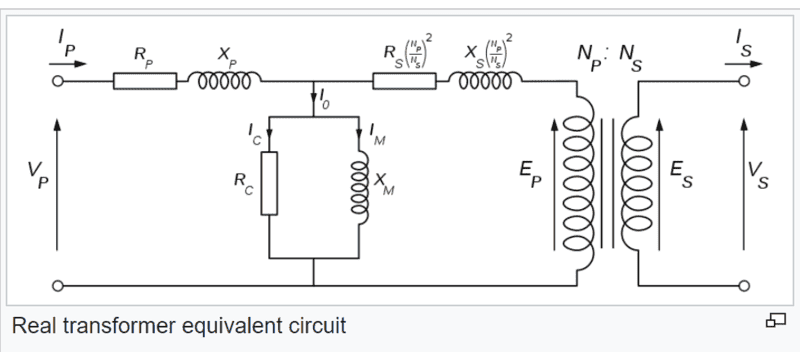I can make you an equivalent circuit of Canada. Would that trigger a discussion of Ohm's Law?

••Tom.G and DaveE
Gold Member
I can make you an equivalent circuit of Canada. Would that trigger a discussion of Ohm's Law?
Yup, probably.

The ideal circuit elements used in network analysis are intentionally defined to be as simple as possible. Boringly simple, and not worth a lot of discussion. How you might choose to use them IRL is a different and infinitely complex subject with no simple answers.

I, for one, am done here. This discussion is pointless.

•phinds
Gold Member
2022 Award
So ... @PhysicsTest the subject has not been beaten to death and then the corpse kicked around some more. Has your question been answered?

PhysicsTest
Yes i think i understood, suppose if i use a voltage source of ##V## volts and resistor ##R## the current
## I ## will flow through resistor ##I = \frac{V} {R} ##.
And if i use a Current source of ## I ## and resistor ##R## then a voltage drop of ##V = IR ## is applied across the resistor.

•Tom.G
Gold Member
2022 Award
Yes, although "applied" isn't really right. If you supply a current of I and have a resistance of R, then a voltage of V=IR is CREATED across R.

•cabraham and berkeman
PhysicsTest
Sorry yes created across the resistor.

•cabraham and phinds
alan123hk
An ideal voltage source is characterized by the equation v(i)=Vo , a constant. Resistors are characterized by v(i)=R⋅i , Ohm's law. If you set R=0 , Ohm's law gives you v(i)=0 . To capture the non-zero source behavior (i.e. the ability to generate power, for example), you would need Ohm's law to look like v(i)=R⋅i+Vo , which it does not. Ohm's law only works for passive devices.

I mean, when doing circuit analysis, Ohm’s law certainly applies to any resistance part inside the voltage source and current source (but even if we agree with this, this may be taken for granted and has no meaning for discussion😓)

In any case, you have explained very clearly that voltage sources and current sources cannot be regarded as resistive elements under Ohm's law, nor are they impedance elements. Of course, I must agree with this.

By the way, I believe that many things we often do in this world may not have much point or practical value. Even if some people think it is a bit meaningful, others may think it is completely meaningless and waste time, but many times we will involuntarily try to continue doing these things.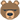Last edited:
Gold Member
You said Ohm's law applies to current sources, but then describe the voltage drop across a resistor? No. An ideal current source has a current defined a priori than does not have any response to the applied voltage.
The sentence you don't like could probably have been written "Ohm's Law applies when using current sources to measure a circuit element". That would sort out who's doing what and to whom.

Ohm's law describes the behaviour of a passive device (in fact just a metal conductor) and not a Power Supply. The relationship
R=V/I
can be shown to be true by varying either V or I and measuring the value of the other one. You can choose to use a constant voltage, constant current or just any old source and V/I will still apply.

Gold Member
The sentence you don't like could probably have been written "Ohm's Law applies when using current sources to measure a circuit element". That would sort out who's doing what and to whom.

Ohm's law describes the behaviour of a passive device (in fact just a metal conductor) and not a Power Supply. The relationship
R=V/I
can be shown to be true by varying either V or I and measuring the value of the other one. You can choose to use a constant voltage, constant current or just any old source and V/I will still apply.
Yes, exactly.

I do think there's a subtle difference though. As you say ohm's law is ##R=V/I##, but a lot of experienced EEs actually work with ##r=\frac{dv}{di}##, the local slope of v(i). This is sometimes more helpful for modelling, but confusing when they talk to beginners.

Last edited:
Gold Member
but a lot of experienced EEs actually work with di/dt
give kids a filament bulb to plot and what can they do?

Gold Member
give kids a filament bulb to plot and what can they do?
I find it quite annoying when intro EE classes use light bulbs in their series/parallel examples. It probably isn't confusing to the students because they don't know better, but it confuses me.

Gold Member
but it confuses me.
I have a feeling that this could be because people say things like:
"Ohm's Law is R=V/I" and "By Ohm's Law".
This is not accurate and not what Ohm said. R is only 'constant' under some conditions. When it isn't constant, the law that R follows may need to be described using the graph slope as well as the ratio (small signal AC behaviour etc).
This is only a problem because of the sloppy way we get taught in the first place. My Mr Scales (A level teacher) never made it a problem.

LvW
Yes, although "applied" isn't really right. If you supply a current of I and have a resistance of R, then a voltage of V=IR is CREATED across R.
This statement sounds as if the voltage across a resistor would be the RESULT of the current through this resistor. Of course, this is NOT true!
A current through a device can only be the result of a voltage across the device (resp. the corresponding E-field within the conducting device).
Yes - during calculation of electrical networks we can use the equation V=I*R (and we may ASSUME that the voltage V is caused by the current I) - but from the physical point of view this would be incorrect.
I think, a good engineer should know how (and why) he is allowed to use some formulas during mathematical manipulations.

Gold Member
This statement sounds as if the voltage across a resistor would be the RESULT of the current through this resistor. Of course, this is NOT true!
Not really "of course". We shouldn't get too hung up about which is the right way to look at things.
Any equation can be re-arranged but the resulting arrangement doesn't imply the 'direction' of cause and effect; it just describes a relationship between two quantities. We are familiar with voltage sources and there is a temptation to make assumptions that it's always the Volts making things happen.

A voltage source has a source of charges at its terminals (perhaps a massive car battery), each of which has potential energy with respect to the other terminal. An easily made system which will keep that Joules per Coulomb relationship over a wide range of current that's passed, maintaining the V marked on the side. I = V/R Amps

But consider a beam of very fast charged particles (electrons would be convenient) that arrives at a cup shaped collector. They arrive at the rate of iCoulombs per second (iA) and will pass through a resistor of value R. They are connected to a conductor, linked to the source of the beam. A voltmeter, connected across the resistor will measure iR Volts.

Both of the above situations involve a source of Power so what's the difference - except that one is much more familiar to us? Potential or kinetic energy - which is the more basic?

•cabraham, alan123hk and Tom.G
Gold Member
This statement sounds as if the voltage across a resistor would be the RESULT of the current through this resistor. Of course, this is NOT true!
A current through a device can only be the result of a voltage across the device (resp. the corresponding E-field within the conducting device).
Yes, but...
Electronic circuits are a pretty artificial approximation of the underlying physics. There really isn't much of a problem with V=I⋅R, I=V/R, or R=V/I. They are all equally effective ways of solving a simplified model of the real world. So, it is common for people to speak in a sloppy fashion about this.

•sophiecentaur
alan123hk
The charge flow must be driven by an electric field. The electric field is caused by the accumulated charge, and the accumulated charge is the voltage. Therefore, the conclusion is that voltage causes current. Voltage is the cause, current is the result. This seems reasonable.

However, some people may ask, since the voltage is caused by the accumulated charge, the accumulated charge is first caused by the moving charge, so if there is no current, the voltage cannot be formed and maintained, so why can't it be said that the current is the cause and the voltage is result.

To be honest, at the moment I am not sure which of these two opinions is correct. I do feel a little confused. I feel that this question is very similar to the question of whether there is a chicken or an egg first. This question is also a bit like when we ask when electromagnetic waves propagate energy, do electric fields appear first or magnetic fields appear first?

So personally, until I find a more convincing and in-depth explanation from the perspective of physics, I dare not draw a conclusion on this issue for the time being.

Gold Member
The error is to assign either as the cause of the other.
We should know better than to ask “what’s really happening?”.

•cabraham, Tom.G, DaveE and 2 others
LvW
However, some people may ask, since the voltage is caused by the accumulated charge, the accumulated charge is first caused by the moving charge, so if there is no current, the voltage cannot be formed and maintained, so why can't it be said that the current is the cause and the voltage is result.
Yes - I agree to your explanation.
However, the remaining question is:
Is the "moving charge" which builds up the voltage equal to the phenomenon we usually call "current"? For example, is the induced voltage in a generator the result of a current?
I doubt - and, therefore, I think that the sequence voltage-current is not a "chicken-egg" problem.

Staff Emeritus
Yes - I agree to your explanation.
However, the remaining question is:
Is the "moving charge" which builds up the voltage equal to the phenomenon we usually call "current"? For example, is the induced voltage in a generator the result of a current?
I doubt - and, therefore, I think that the sequence voltage-current is not a "chicken-egg" problem.
You have to use Maxwell's Equations, or QED, to get the "real" answer. Circuit analysis, and thinking with circuits won't get you there.

•cabraham, DaveE and sophiecentaur
Gold Member
Yes i think i understood, suppose if i use a voltage source of ##V## volts and resistor ##R## the current
## I ## will flow through resistor ##I = \frac{V} {R} ##.
And if i use a Current source of ## I ## and resistor ##R## then a voltage drop of ##V = IR ## is applied across the resistor.
Sure. However, if there is a ground fault or disconnect from GND in the BJT example of a current source, or any current source for that matter, the transistor/source will shutoff.